• 主要介绍了matlab中二维插值函数interp2的使用详解，具有很好的参考价值，希望对大家有所帮助。一起跟随小编过来看看吧
• 主要介绍了Numpy一维线性插值函数的用法，具有很好的参考价值，希望对大家有所帮助。一起跟随小编过来看看吧
• 编写程序构造区间上的以等分结点为插值结点的Newton插值公式假设结点数为包括两个端点给定相应的函数值插值区间和等分的份数该程序能快速计算...不同的值并画出原函数的图像以及插值函数的图像观察当增大时的逼近效果....
• 已知一组数据点 编写一程序求解三次样 条插值函数 满足 并针对下面一组具体实验数据 0.25 0.3 0.39 0.45 0.53 0.5000 0.5477 0.6245 0.6708 0.7280 求解其中边界条件为 . 解 Matlab 计算程序为 clear clc x=[0.25 ...
• 样条插值函数 matlab的spline函数的c语言实现版 支持3点及以上插值 效果超好
• 对三次样条插值函数的m文件，下载可直接可用，用于matlab编程设计和课程作用 对三次样条插值函数的m文件，下载可直接可用，用于matlab编程设计和课程作用
• 利用python中matplotlib库，实现绘制牛顿插值、拉格朗日插值、三次样条插值函数图像。 随机在图像上产生5个随机点，用三种插值方式求函数，并用python中matplotlib库绘制在图像上
• 有限元基础(单元和插值函数的构造)建立基本的有限元概念
• ## Scipy插值函数

千次阅读 2020-05-30 11:51:39
Scipy插值函数导入插值模块一维插值函数interp1d二维插值函数样条插值函数 在机器人控制控制过程中，当我们规划频率太低（规划的点时间间隔太大），而控制底层需要较高的控制频率，这时往往通过插值的方式实现低频率...


Scipy插值函数
导入插值模块一维插值函数interp1d二维插值函数样条插值函数

在机器人控制控制过程中，当我们规划频率太低（规划的点时间间隔太大），而控制底层需要较高的控制频率，这时往往通过插值的方式实现低频率到高频率的转换。常用的插值函数有线性插值、梯形插值、三次多项式插值、五次多项式插值、拉格朗日插值、三次样条插值等，其中常用的是三次样条插值和五次多项式插值。自己编写插值函数难度也不是特别大，我将在“协作机器人控制栏”如何自己编写插值函数，但是对于小白或想快速编写插值函数，我们可以直接调用Scipy中的插值模块实现。

导入插值模块
可以通过下面方法导入插值模块
#导入插值模块
from scipy import interpolate

通过查询interpolate/interpolate.py文件，可以知道插值函数包括一维插值、二维插值、样条插值等
__all__ = ['interp1d', 'interp2d', 'spline', 'spleval', 'splmake', 'spltopp',
'ppform', 'lagrange', 'PPoly', 'BPoly', 'RegularGridInterpolator',
'interpn']

一维插值函数interp1d
使用interp1d一维插值函数是指单变量函数，给定一维自变量x和因变量y，创建一个连续函数f,通过函数f(new_x)求取函数中对应的数值点。
y1 = interp1d(x, y, kind='linear')

线面是代码实现
#!/usr/bin/env python
#-*-coding:utf-8-*-
#本文档用于scipy学习
#程序员：飞龙
#版权：哈尔滨工业大学(深圳)
#日期：2020年5月30号

import numpy as np
import matplotlib.pyplot as plt

#导入插值模块
from scipy.interpolate import interp1d

#生成数据
x = np.linspace(0, 1, 30)
y = np.sin(5*x) + np.cos(10*x)

#**一维插值函数***#
#零次插值
y0 = interp1d(x, y, kind='zero')
#一次插值
y1 = interp1d(x, y, kind='linear')
#二次插值
#三次插值
y3 = interp1d(x, y, kind='cubic')

#新变量
new_x = np.linspace(0, 1, 100)
#绘图
plt.figure()
plt.plot(x, y, 'o', label='data')
plt.plot(new_x, y0(new_x), label='zero')
plt.plot(new_x, y1(new_x), label='linear')
plt.plot(new_x, y3(new_x), label='cubic')
plt.title("interp1d")
plt.xlabel("x")
plt.ylabel("f(x)")
plt.legend()
plt.show()

运行结果：二维插值函数
Scipy还提供了二维插值函数interp2d,该函数能实现二维插值，其调用格式如下
#一次插值
z1 = interp2d(x, y, z, kind='linear')
new_z1 = z1(new_x, new_x)

其中第一、二个参数是一维数组，其中x为m维，为n维，第三个参数z是n*m二维数组，第四个参数为插值类型。返回一个连续插值函数，通过输入新的插值点实现调用。
完整代码实现
#!/usr/bin/env python
#-*-coding:utf-8-*-
#本文档用于scipy学习
#程序员：陈永厅
#版权：哈尔滨工业大学(深圳)
#日期：2020年5月30号

import numpy as np
import matplotlib.pyplot as plt

#导入插值模块
from scipy.interpolate import interp2d

#生成数据
x = np.linspace(0, 1, 20)
y = np.linspace(0, 1, 30)
xx, yy = np.meshgrid(x, y)
rand = np.random.rand(600).reshape([30, 20])
z = np.sin(xx**2) + np.cos(yy**2) + rand

new_x = np.linspace(0, 1, 100)
new_y = np.linspace(0, 1, 100)

#**样条插值函数插值函数***#
#一次插值
z1 = interp2d(x, y, z, kind='linear')
new_z1 = z1(new_x, new_y)

#三次插值
z3 = interp2d(x, y, z, kind='cubic')
new_z3 = z3(new_x, new_y)

#绘图
plt.figure()
plt.plot(x, z[0, :], 'o', label='data')
plt.plot(new_x, new_z1[0, :], label='linear')
plt.plot(new_x, new_z3[0, :], label='cubic')
plt.title("interp2d")
plt.xlabel("x")
plt.ylabel("f")
plt.legend()
plt.show()

#用矩阵显示z
plt.matshow(z)
plt.title("data")
plt.xlabel("x")
plt.ylabel("y")
plt.show()

#用矩阵显示z
plt.matshow(new_z1)
plt.title("linear")
plt.xlabel("x")
plt.ylabel("y")
plt.show()

#用矩阵显示z
plt.matshow(new_z3)
plt.title("cubic")
plt.xlabel("x")
plt.ylabel("y")
plt.show()

输出结果图： 为了在同一幅图中比较两种插值效果，下图取其中一维在二维图中显示要想更直观显示二维插值与一维插值的区别，本文采用矩阵显示的方法显示插值结果如下 图1原始数据图图2线性插值图图3 三次插值图样条插值函数
样条曲线(Spline)是早起工程绘图中使用的一种工具，是富有弹性的细木条和金属条，利用他可以将一系列离散点连接成光滑曲线，称为样条曲线，后来数学家将其抽象，定义了样条函数，其中常用的是三次样条曲线，由分段三次多项式组成，在连接点具有连续曲率。
y3 = spline(x, y, new_x, order=3, kind='smoothest')

调用格式，第一参数原始自变量，第二个参数原始因变量，第三个参数需要插值的点，第四个参数样条阶数，为整数，第五个参数样条类型。 完整代码如下：
#!/usr/bin/env python
#-*-coding:utf-8-*-
#本文档用于scipy学习
#程序员：陈永厅
#版权：哈尔滨工业大学(深圳)
#日期：2020年5月30号

import numpy as np
import matplotlib.pyplot as plt

#导入插值模块
from scipy.interpolate import spline

#生成数据
x = np.linspace(0, 1, 30)
y = np.sin(5*x) + np.cos(10*x) + np.random.rand(30)
new_x = np.linspace(0, 1, 100)

#**样条插值函数插值函数***#
#零次插值
y0 = spline(x, y, new_x, order=0, kind='smoothest')
#一次插值
y1 = spline(x, y, new_x, order=1, kind='smoothest')
#二次插值
y2 = spline(x, y, new_x, order=2, kind='smoothest')
#三次插值
y3 = spline(x, y, new_x, order=3, kind='smoothest')

#绘图
plt.figure()
plt.plot(x, y, 'o', label='data')
plt.plot(new_x, y0, label='zero')
plt.plot(new_x, y1, label='linear')
plt.plot(new_x, y3, label='cubic')
plt.title("spline")
plt.xlabel("x")
plt.ylabel("f")
plt.legend()
plt.show()

运行结果展开全文python
• 将三次样条函数封装成了一个CSpline类。实现了样条函数I型边界条件和II型边界条件。
• 用Visual Studio 2012 实现了三次样条插值函数。概述如下： 主要数据结构：typedef vector<double> VECTOR 三次样条函数：VECTOR Spline(VECTOR Node, VECTOR Value, VECTOR x0, double c1, double c2, int ...
• C#实现抛物线插值函数 using System; using System.Collections.Generic; using System.ComponentModel; using System.Data; using System.Drawing; using System.Linq; using System.Text; using System.Windows....
• Matlab中插值函数汇总和使用说明 关于一些基本的源程序
• MATLAB中的插值函数为interp1，其调用格式为：yi= interp1(x,y,xi,'method')， 其中x，y为初始插值点，xi为给定的插值点，yi为在被插值点xi处的插值结果。 'method'表示采用的插值方法，MATLAB提供的插值方法有...
一、interp1
1、函数简介
MATLAB中的插值函数为interp1，其调用格式为：yi= interp1(x,y,xi,'method')，
其中x，y为初始插值点，xi为给定的插值点，yi为在被插值点xi处的插值结果。
'method'表示采用的插值方法，MATLAB提供的插值方法有几种：    'nearest'是最邻近插值， 'linear'线性插值； 'spline'三次样条插值； 'pchip'立方插值．缺省时表示线性插值    注意：所有的插值方法都要求x是单调的，并且xi不能够超过x的范围。
2、用法示例
% 绘制散点图
clc
clear
close
tem=[2 2 0 2 2 2 2 3 5 7 9 10 11 12 12 11 10 9 7 7 6 6 5 5];
time=1:24;
plot(time,tem,'o')
hold on

% 第一种用法
xq=1:0.1:24;
vq=interp1(time,tem,xq);
plot(xq,vq) %绘制插值曲线
close
%第二种用法
% linear  线形（默认）
% nearest 临近点
% next    下一个
% previous 前一个
% pchip 保形分段三次插值
% cubic
% v5cubic
% spline  三次方样条数据插值

% 线性
subplot(2,3,1)
xq=1:0.5:24;
vq=interp1(time,tem,xq,'linear');
plot(xq,vq,'*')
title('linear')

% 临近点
subplot(2,3,2)
xq=1:0.5:24;
vq=interp1(time,tem,xq,'nearest');
plot(xq,vq,'*')
title('nearest')

% next
subplot(2,3,3)
xq=1:0.5:24;
vq=interp1(time,tem,xq,'next');
plot(xq,vq,'*')
title('next')

% previous
subplot(2,3,4)
xq=1:0.5:24;
vq=interp1(time,tem,xq,'previous');
plot(xq,vq,'*')
title('previous')

% pchip
subplot(2,3,5)
xq=1:0.5:24;
vq=interp1(time,tem,xq,'pchip');
plot(xq,vq,'*')
title('pchip')

% spline
subplot(2,3,6)
xq=1:0.5:24;
vq=interp1(time,tem,xq,'spline');
plot(xq,vq,'*')
title('spline');
close
% 第三种 取出插值多项式的系数
%v = ppval(pp,xq) 在查询点 xq 处计算分段多项式 pp。
x=0:0.5:2*pi;
y=sin(x);
p=interp1(x,y,'spline','pp'); %% 返回各个分段插值多项式p的系数
yy=ppval(p,linspace(0,2*pi,101));
plot(x,y,'o',linspace(0,2*pi,101),yy);
% 第四种
x=0:0.5:2*pi;
y=sin(x);
xi=-1:0.2:7;
p=interp1(x,y,xi,'spline') ;%插值区间xi超出样本区间x自动外推
p=interp1(x,y,xi,'linear','extrap'); %加上'extrap'自动外推
plot(x,y,'o',xi,p);
p=interp1(x,y,xi,'next','extrap'); %加上'extrap'自动外推
p=interp1(x,y,xi,'nearest',9);%令超出范围的数值为9
二、spline三次样条插值
1、函数简介
MATLAB中的插值函数为interp1，其调用格式为：yi= spline(x,y,xi)，
其中x，y为初始插值点，xi为给定的插值点，yi为在被插值点xi处的插值结果。
2、用法示例
%第一种 单条曲线插值
x=0:0.5:2*pi;
y=sin(x);
xx=0:0.1:2*pi;
yy=spline(x,y,xx);
plot(x,y,'o',xx,yy,'-')
close

% 第二种 多条曲线插值
x=0:0.5:2*pi;
y=[sin(x);cos(x)];
xx=0:0.1:2*pi;
yy=spline(x,y,xx);
plot(x,y,'o',xx,yy,'-')

% 第三种 取出插值多项式的系数
x=0:0.5:2*pi;
y=sin(x);
p=spline(x,y); %返回各个分段插值多项式p的系数
xx=0:0.1:2*pi;
yy=ppval(p,xx); %v = ppval(pp,xq) 在查询点 xx 处计算分段多项式 p。
plot(x,y,'o',xx,yy) 
三、pchip 分段三次 Hermite 插值多项式
1、函数简介
其函数使用方法跟以上的类似，我们这里直接上代码。
2、函数代码
%第一种
x=0:0.5:2*pi;
y=sin(x);
xx=0:0.1:2*pi;
yy=pchip(x,y,xx);
plot(x,y,'o',xx,yy,'-')
% 第二种 取出插值多项式的系数
x=0:0.5:2*pi;
y=sin(x);
p=pchip(x,y);
xx=0:0.1:2*pi;
yy=ppval(p,xx); %v = ppval(pp,xq) 在查询点 xx 处计算分段多项式 pp。
plot(x,y,'o',xx,yy)

展开全文matlab 算法
• 四点插值函数，用于补出测量空缺部分的函数值。内插结果较为准确，外插结果不准
• 插值法 插值法又称“内插法”，是利用函数f (x)在某区间中已知的若干点的函数值，作出适当的特定函数，在区间的其他点上用这特定函数的值作为函数f (x)的近似值，这种方法称为插值法。如果这特定函数是多项式，就称...
插值法
插值法又称“内插法”，是利用函数f (x)在某区间中已知的若干点的函数值，作出适当的特定函数，在区间的其他点上用这特定函数的值作为函数f (x)的近似值，这种方法称为插值法。如果这特定函数是多项式，就称它为插值多项式。

线性插值法
线性插值法是指使用连接两个已知量的直线来确定在这两个已知量之间的一个未知量的值的方法。
假设我们已知坐标(x0,y0)与(x1,y1),要得到[x0,x1]区间内某一位置x在直线上的值。根据图中所示，我们得到两点式直线方程：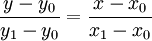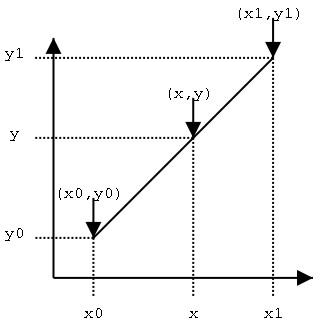假设方程两边的值为α，那么这个值就是插值系数—从x0到x的距离与从x0到x1距离的比值。由于x值已知，所以可以从公式得到α的值：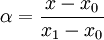同样：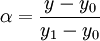这样，在代数上就可以表示成为：
y = (1 − α)y0 + αy1
或者，
y = y0 + α(y1 − y0)
这样通过α就可以直接得到 y。实际上，即使x不在x0到x1之间并且α也不是介于0到1之间，这个公式也是成立的。在这种情况下，这种方法叫作线性外插—参见 外插值。
已知y求x的过程与以上过程相同，只是x与y要进行交换。
MATLAB中的使用

[plain]
view plain
copy

%{      MATLAB中的插值函数为interp1，其调用格式为：  yi= interp1(x,y,xi,'method')                 其中x，y为插值点，yi为在被插值点xi处的插值结果；x,y为向量，       'method'表示采用的插值方法，MATLAB提供的插值方法有几种：           'nearest'是最邻近插值， 'linear'线性插值； 'spline'三次样条插值； 'pchip'立方插值．缺省时表示线性插值      注意：所有的插值方法都要求x是单调的，并且xi不能够超过x的范围。  %}  x = 0:2*pi;    y = sin(x);    xx = 0:0.5:2*pi;      % interp1对sin函数进行分段线性插值，调用interp1的时候，默认的是分段线性插值    y1 = interp1(x,y,xx,'linear');    subplot(2,2,1);  plot(x,y,'o',xx,y1,'r')    title('分段线性插值')        % 临近插值    y2 = interp1(x,y,xx,'nearest');    subplot(2,2,2);  plot(x,y,'o',xx,y2,'r');    title('临近插值')        %球面线性插值    y3 = interp1(x,y,xx,'spline');    subplot(2,2,3);  plot(x,y,'o',xx,y3,'r')    title('球面插值')        %三次多项式插值法    y4 = interp1(x,y,xx,'pchip');    subplot(2,2,4);  plot(x,y,'o',xx,y4,'r');    title('三次多项式插值')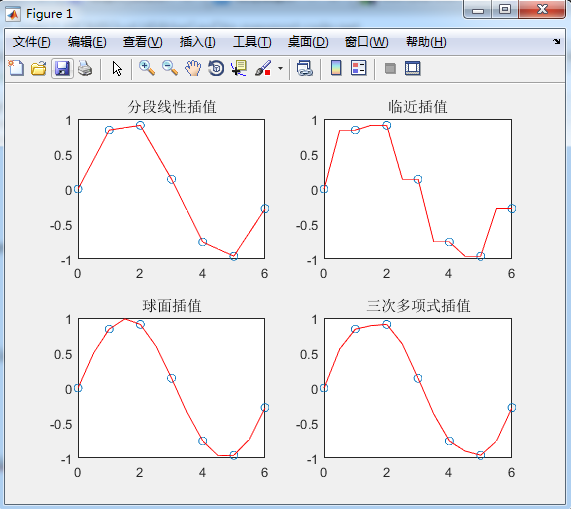一个例子

[plain]
view plain
copy

%{      MATLAB中的插值函数为interp1，其调用格式为：  yi= interp1(x,y,xi,'method')                 其中x，y为插值点，yi为在被插值点xi处的插值结果；x,y为向量，       'method'表示采用的插值方法，MATLAB提供的插值方法有几种：           'nearest'是最邻近插值， 'linear'线性插值； 'spline'三次样条插值； 'pchip'立方插值．缺省时表示线性插值      注意：所有的插值方法都要求x是单调的，并且xi不能够超过x的范围。  %}    %{      例如：在一 天24小时内，从零点开始每间隔2小时测得的环境温度数据分别为              12，9，9，1，0，18 ，24，28，27，25，20，18，15，13，      推测中午12点（即13点）时的温度．  %}  x = 0:2:24;  y = [12   9   9   10   18  24   28   27   25   20  18  15  13];  a = 13;  y1 = interp1(x,y,a,'spline')  % 结果为：  27.8725    % 若要得到一天24小时的温度曲线，则：  xi = 0:1/3600:24;  % 插值点可以是向量，则返回的也就是对应的向量  yi = interp1(x,y,xi, 'spline');  plot(x,y,'o' ,xi,yi);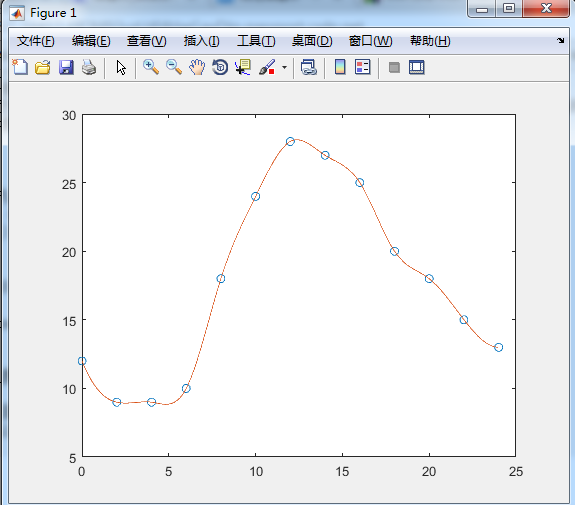[plain]
view plain
copy

x=0:2*pi;  y=sin(x);  xx=0:0.5:2*pi;    %interp1对sin函数进行分段线性插值，调用interp1的时候，默认的是分段线性插值  y1=interp1(x,y,xx);  figure  plot(x,y,'o',xx,y1,'r')  title('分段线性插值')    %临近插值  y2=interp1(x,y,xx,'nearest');  figure  plot(x,y,'o',xx,y2,'r');  title('临近插值')    %球面线性插值  y3=interp1(x,y,xx,'spline');  figure  plot(x,y,'o',xx,y3,'r')  title('球面插值')    %三次多项式插值法  y4=interp1(x,y,xx,'cubic');  figure  plot(x,y,'o',xx,y4,'r');  title('三次多项式插值')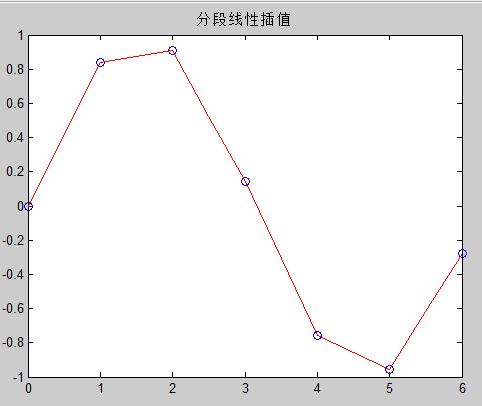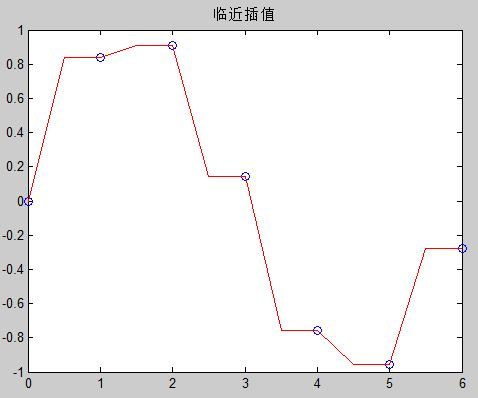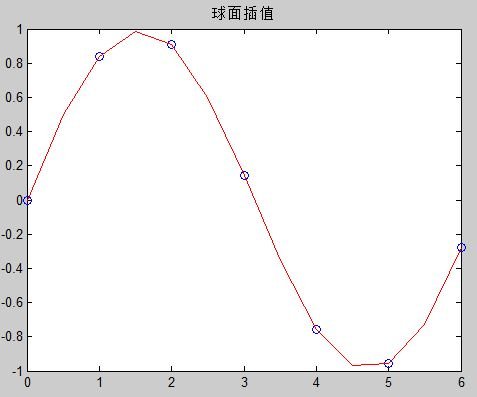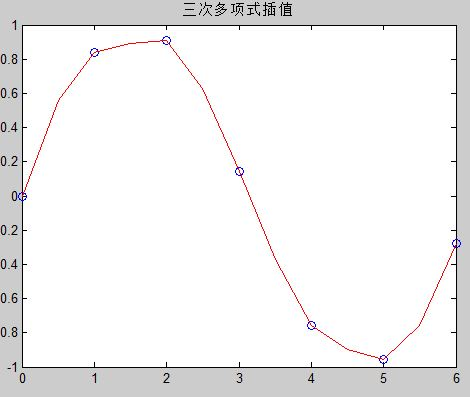(1)    Nearest方法速度最快，占用内存最小，但一般来说误差最大，插值结果最不光滑。
(2)    Spline三次样条插值是所有插值方法中运行耗时最长的，插值函数及其一二阶导函数都连续，是最光滑的插值方法。占用内存比cubic方法小，但是已知数据分布不均匀的时候可能出现异常结果。
(3)    Cubic三次多项式插值法中，插值函数及其一阶导数都是连续的，所以插值结果比较光滑，速度比Spline快，但是占用内存最多。
语法形式说明y=interp1(x,Y,xi)由已知点集(x,Y)插值计算xi上的函数值y=interp1(x,Y,xi)相当于x=1:length(Y)的interp(x,Y,xi)y=interp1(x,Y,xi,method)用指定插值方法计算插值点xi上的函数值y=interp1(x,Y,xi,method,’extrap’)对xi中超出已知点集的插值点用指定插值方法计算函数值y=interp1(x,Y,xi,method,’extrap’,extrapval)用指定方法插值xi上的函数值，超出已知点集处函数值取extrapvaly=interp1(x,Y,xi,method,’pp’)用指定方法插值，但返回结果为分段多项式

Method方法描述‘nearest’最邻近插值:插值点处函数值与插值点最邻近的已知点函数值相等‘liner’分段线性插值：插值点处函数值由连接其最邻近的两侧点的线性函数预测。Matlab中interp1的默认方法。‘spline’样条插值：默认为三次样条插值。可用spline函数替代‘pchip’三次Hermite多项式插值，可用pchip函数替代‘cubic’同’pchip’，三次Hermite多项式插值

另：

【matlab】csape 和interp1有什么相同和区别？

csape和interp1都是插值函数。csape可以选择样条的边界条件，interp1无法使用边界条件；csape只是Cubic spline插值，interp1可以选择几种不同的插值方法。csape函数的用法如下：pp = csape(x,y,conds,valconds)其中(x,y)为数据向量，conds表示变界类型， valconds表示边界值。边界类型(conds)可为：'complete'，给定边界一阶导数. 'not-a-knot'，非扭结条件,不用给边界值. 'periodic'，周期性边界条件,不用给边界值. 'second'，给定边界二阶导数.  'variational'，自然样条(边界二阶导数为0)边界类型(valconds)可为：'complete'，给定边界一阶导数.'not-a-knot'，非扭结条件,不用给边界值.'periodic'，周期性边界条件,不用给边界值.'second'，给定边界二阶导数. 'variational'，自然样条(边界二阶导数为0)interp1函数的用法如下：　　yi=interp1(x,Y,xi)：返回插值向量yi，每一元素对应于参量xi，同时由向量X与Y的内插值决定。参量x 指定数据Y的点。若Y为一矩阵，则按Y的每列计算。yi是阶数为length(xi)*size(Y,2)的输出矩阵。　　yi=interp1(Y,xi)：假定x=1:N，其中N为向量Y的长度，或者为矩阵Y的行数。　　yi=interp1(x,Y,xi,method)：用指定的算法计算插值。nearest为最近邻点插值，直接完成计算；linear为线性插值（默认方式），直接完成计算；spline为三次样条函数插值。　　yi=interp1(x,Y,xi,method,'extrap')：对于超出x范围的xi中的分量将执行特殊的外插值法extrap。　　yi=interp1(x,Y,xi,method,extrapval)：确定超出x范围的xi中的分量的外插值extrapval，其值通常取NaN或0。

展开全文matlab
• 数值计算方法--插值函数(java实现) 数值计算方法--插值函数(java实现) 数值计算方法--插值函数(java实现) 数值计算方法--插值函数(java实现) 数值计算方法--插值函数(java实现) 数值计算方法--插值函数(java实现) ...
• 对给定的点进行三次B样条插值，得到插值曲线，这里给定的点可以是二维平面上的点或三维点，注意输入的点矩阵要每行为一个点坐标，里面都有注释，可以自己简单修改封装成自己想要的带参函数，里面有测试的点数据，...
• ## python scipy 插值函数

千次阅读 2020-07-30 10:55:48
python scipy 插值函数 """ 数据补全 插值 根据离散散点 (使用不同的插值方式) 线性，三次样条等 生成不同的 插值函数 用来获取连续的点 """ import numpy as np import scipy.interpolate as si import ...
python scipy 插值函数
"""
数据补全 插值
根据离散散点  (使用不同的插值方式) 线性，三次样条等
生成不同的 插值函数 用来获取连续的点
"""
import numpy as np
import scipy.interpolate as si
import matplotlib.pyplot as mp

# 准备数据
min_x = -50
max_x = 50
x = np.linspace(min_x, max_x, 17)
y = np.sinc(x)

# 绘制数据
mp.grid(linestyle=":")
mp.scatter(x, y, s=60, color="dodgerblue",
marker="o", label="samples")

# 通过样本点 ==>  插值函数(线性)
linear = si.interp1d(x, y, kind="linear")

linear_x = np.linspace(min_x, max_x, 1000)
linear_y = linear(linear_x)
mp.plot(linear_x, linear_y, color="g",
label="linear interp1d")

# 通过样本点 ==>  插值函数(三次样条)
cubic = si.interp1d(x, y, kind="cubic")

cubic_y = cubic(linear_x)
mp.plot(linear_x, cubic_y, color="r",
label="cubic interp1d")

mp.legend()
mp.show()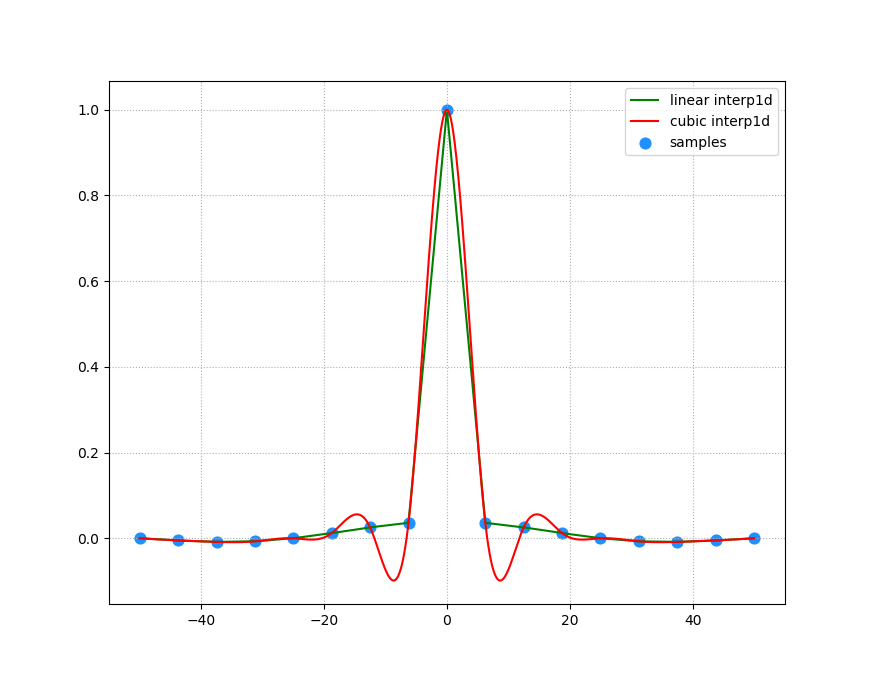展开全文• interpl( )一维插值函数 格式： yi＝interpl (x, y, xi, 'method') 功能：为给定的数据对(x,y)以及x坐标上的插值范围向量xi，用指定所使用的插值方法method实现插值。yi是插值后的对应数据点集的y坐标 简单来...interp1 interp2 matlab 线性插值
• ## Matlab中插值函数汇总及使用说明

万次阅读 多人点赞 2018-09-12 19:44:52
MATLAB中的插值函数为interp1，其调用格式为： yi= interp1(x,y,xi,'method')  其中x，y为插值点，yi为在被插值点xi处的插值结果；x,y为向量， 'method'表示采用的插值方法，MATLAB提供的插...
• 自己用matlab编写的3次样条插值函数,绝对原创.
• 问题 已知 X和Y有下表的对应关系，当一个新的4<...采用线性插值的方法，找到x前后两个数x1和x2，线性计算 y=y1 + x*(y2-y1)/(x2-x1)即可 因此 x=10，y应该是85 Excel函数介绍 trend（）函数 说明 返...excel 算法
• 泛函的一般形式是函数的积分，有限元法是求解泛函极值的一种方法。 在有限元法的计算过程中，会遇到大量积分计算，用一般的方法计算积分，常常很麻烦。为了简化这些计算，引入自然坐标和等参单元。 自然坐标 有限元...
• function yy=lagrange(x,y,xx) %号Lagrange插值，求数据（x，y）所表达的函数插值点xx处的插值 m=length(x); n=length(y); if m~=n,error (‘向量x与y的长度必须一致’) end s=0; for i=1:n t=ones(1,length(xx));...matlab
• matlab三次样条插值函数代码三次样条插值 这是一个函数，用于显示插值的工作方式以及如何将MATLAB inperp2（'spline'）转换为C ++。 关于三次样条的重要说明 当指定样条标记时，MATLAB的interp1假定端点条件不是knot......